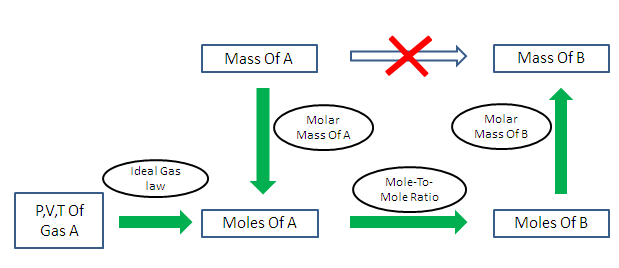# Amount of substance (n) Calculation

The calculator calculates the amount of substance having the number of molecules, ions, atoms or other specified group of entities present in any compound, which is the ratio of Mass / Molar mass.
The mole is fundamental unit in the SI system and it is used to measure the amount of substance.

#### Formula Used:

Amount of substance (n)=Mass (m)/Molar mass (M)

Mass is the weight in kilograms, whereas molarmass is the mass of one mole of a compound in g/mol(gram/mole).

 Mass(kg) : Molar Mass(g/mol): Amount of Substance (n) : (mole)